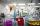# Substitution method

Solve goniometric equation:

sin4 θ - 1/cos2 θ=cos2 θ - 2

Result

θ = (Correct answer is: k*pi)#### Solution:Leave us a comment of example and its solution (i.e. if it is still somewhat unclear...):

Showing 0 comments:Be the first to comment!#### To solve this example are needed these knowledge from mathematics:

Looking for help with calculating roots of a quadratic equation? Do you have a linear equation or system of equations and looking for its solution? Or do you have quadratic equation? Most natural application of trigonometry and trigonometric functions is a calculation of the triangles. Common and less common calculations of different types of triangles offers our triangle calculator. Word trigonometry comes from Greek and literally means triangle calculation.

## Next similar examples:

1. Completing squareSolve the quadratic equation: m2=4m+20 using completing the square method
2. Solve 3Solve quadratic equation: (6n+1) (4n-1) = 3n2
3. Solve equationsolve equation: ?
4. Reciprocal equation 2Solve this equation: x + 5/x - 6 = 4/11
5. RootsDetermine the quadratic equation absolute coefficient q, that the equation has a real double root and the root x calculate: ?
6. Quadratic equationFind the roots of the quadratic equation: 3x2-4x + (-4) = 0.
7. Square root 2If the square root of 3m2 +22 and -x = 0, and x=7, what is m?
8. Evaluation of expressionsIf a2-3a+1=0, find (i)a2+1/a2 (ii) a3+1/a3
9. EquationEquation ? has one root x1 = 8. Determine the coefficient b and the second root x2.
10. DiscriminantDetermine the discriminant of the equation: ?
11. Quadratic equationQuadratic equation ? has roots x1 = 80 and x2 = 78. Calculate the coefficients b and c.
12. Crystal waterThe chemist wanted to check the content of water of crystallization of chromic potassium alum K2SO4 * Cr2 (SO4) 3 * 24 H2O, which was a long time in the laboratory. From 96.8 g of K2SO4 * Cr2 (SO4) 3 * 24 H2O prepared 979 cm3 solution of base. S
13. Difference AP 4Calculate the difference of the AP if a1 = 0.5, a2 + a3 = -1.1
14. AlgebraX+y=5, find xy (find the product of x and y if x+y = 5)
15. Cinema 4In cinema are 1656 seats and in the last row are 105 seats , in each next row 3 seats less. How many are the total rows in cinema?
16. Theorem proveWe want to prove the sentense: If the natural number n is divisible by six, then n is divisible by three. From what assumption we started?
17. Find the 2Find the term independent of x in the expansion of (4x3+1/2x)8